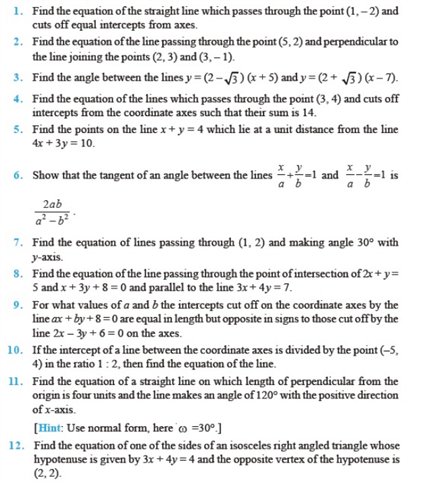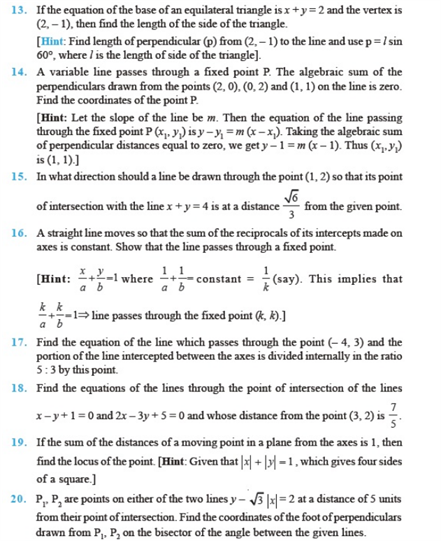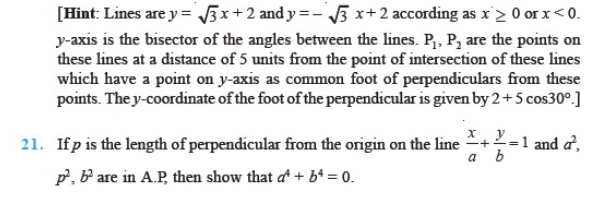# Chapter 10 - Straight lines

Some of the most important Straight Lines Class 11 questions i.e chapter 10 are given here. These chapter 10 class 11 maths questions cover several short answer type questions, long answer type questions and HOTS questions that are crucial for CBSE class 11 exams. Here, some of the important straight lines class 11 questions from NCERT are also included.

Also, Check:

## Straight Lines Important Questions For Class 11 (Chapter 10)

These class 11 Straight Lines questions are categorized into short answer type questions and long answer type questions. These questions cover various concepts which will help class 11 students to develop problem-solving skills for the exam.

1. If the slopes of the two lines are ½ and 3, then find the angle between the two lines.
2. Find the angle between the x-axis and the line joining the points (3, -1) and (4, -2).
3. Find the equations to the line parallel to the axes and passing through the point (-3, 5).
4. Find the equation of the line which is passing through the point (-2, -4) and perpendicular to the line 3x – y + 5 = 0.
5. Find the equation of the line which passes through the point(3,4) and the sum of whose intercepts on the axes is 14.
6. Find the distance of the point (-1,1) from the line 12(x+6) = 5(y-2)
7. Find the point of intersection of the ines 5a
+ 7b = 3 and 2a  – 3b =7.
8. At what point is the origin be shifted, if the coordinates of the point (,5)become (3,7)?
9. Find the area of the triangle formed by the lines y – x = 0, x + y = 0 and x – c= 0.
10. Find the intercepts cut off by the line 2a – b + 16 = 0

### NCERT Class 11 Straight Lines Questions